# The MDC Procedure

### Mixed Logit Model

In mixed logit models, an individual’s utility from any alternative can be decomposed into a deterministic component,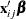, which is a linear combination of observed variables, and a stochastic component,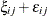,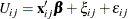where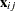is a vector of observed variables that relate to individual i and alternative j,is a vector of parameters,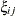is an error component that can be correlated among alternatives and heteroscedastic for each individual, and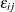is a random term with zero mean that is independently and identically distributed over alternatives and individuals. The conditional logit model is derived if you assumehas an iid Gumbel distribution and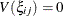.

The mixed logit model assumes a general distribution forand an iid Gumbel distribution for. Denote the density function of the error componentas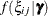, where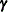is a parameter vector of the distribution of. The choice probability of alternative j for individual i is written as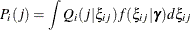where the conditional choice probability for a given value ofis the logit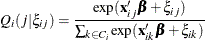Sinceis not given, the unconditional choice probability,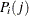, is the integral of the conditional choice probability,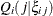, over the distribution of. This model is called "mixed logit" since the choice probability is a mixture of logits withas the mixing distribution.

In general, the mixed logit model does not have an exact likelihood function because the probabilitydoes not always have a closed form solution. Therefore, a simulation method is used for computing the approximate probability,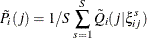where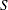is the number of simulation replications and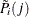is a simulated probability. The simulated log-likelihood function is computed as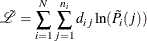where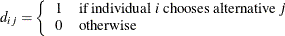For simulation purposes, assume that the error component has a specific structure,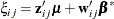where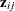is a vector of observed data and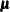is a random vector with zero mean and density function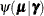. The observed data vector () of the error component can contain some or all elements of. The component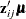induces heteroscedasticity and correlation across unobserved utility components of the alternatives. This allows flexible substitution patterns among the alternatives. The kth element of vectoris distributed as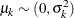Therefore,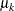can be specified as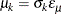where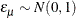or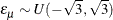In addition,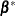is a vector of random parameters (random coefficients). Random coefficients allow heterogeneity across individuals in their sensitivity to observed exogenous variables. The observed data vector,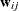, is a subset of. The following three types of distributions for the random coefficients are supported, where the mth element ofis denoted as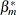:

• Normally distributed coefficient with the mean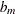and spread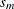being estimated.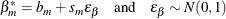• Uniformly distributed coefficient with the meanand spreadbeing estimated. A uniform distribution with mean b and spread s is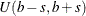.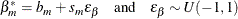• Lognormally distributed coefficient. The coefficient is calculated as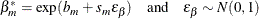where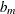andare parameters that are estimated.

The estimate of spread for normally, uniformly, and lognormally distributed coefficients can be negative. The absolute value of the estimated spread can be interpreted as an estimate of standard deviation for normally distributed coefficients.

A detailed description of mixed logit models can be found, for example, in Brownstone and Train (1999).